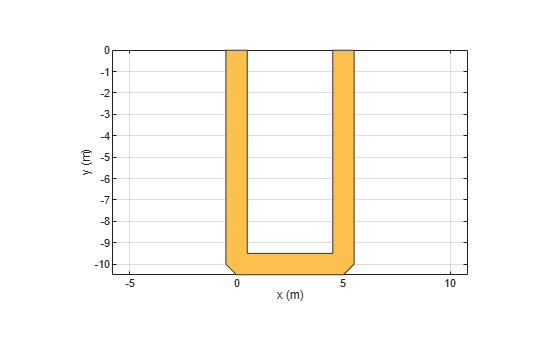# traceLine

Create line trace

## Description

Use the `traceLine` object to create a line trace. You can use this object to create lines of different lengths and different angles

## Creation

### Syntax

``trace = traceLine``
``trace = traceLine(Name=Value)``

### Description

example

````trace = traceLine` creates a line trace using default properties.```

example

````trace = traceLine(Name=Value)` sets properties using one or more name-value arguments. For example, `traceLine('StartPoint',[1 1])` creates a line trace shape with the starting point of `[1 1]`. Properties not specified retain their default values.```

## Properties

expand all

Name of the line trace, specified as a character vector or string scalar.

Example: `customtrace = traceLine(Name=traceline1)`

Data Types: `char` | `string`

Start point of the line trace in Cartesian coordinates, specified as a two-element vector.

Example: `customtrace = traceLine(StartPoint=[1 1])`

Data Types: `double`

Length of line trace, specified as an n-by-1 vector in meters. Each element represents the length of a line segment.

Example: ```customtrace = traceLine(Length=[0.0100 0.0100 0.0100 0.0500])```

Data Types: `double`

Width of the line trace, specified as a scalar or an n-by-1 vector in meters. Each element represents the length of a line segment

Example: ```customtrace = traceLine(Width=[0.0040 0.0040 0.0040 0.0050])```

Data Types: `double`

Angle of the line trace, specified as an n-by-1 vector in degrees. Each element represents an angle of a line segment.

Example: `customtrace = traceLine(Angle=[40 10 -40 35)`

Data Types: `double`

Corner where two line segments interface, specified as either `"Sharp"`, `"Miter"`, or `"Smooth"`. To apply the same value to all corners, specify a string scalar. For a different value for all corners, specify a (n-2)-by-1 vector of strings.

Example: `trace = traceLine(Corner="Miter")`

Data Types: `string`

## Object Functions

 `add` Boolean unite operation on two RF PCB shapes `and` Shape1 & Shape2 for RF PCB shapes `area` Calculate area of RF PCB shape in square meters `intersect` Boolean intersection operation on two RF PCB shapes `mesh` Change and view mesh properties of metal or dielectric in PCB component `minus` Shape1 - Shape2 for RF PCB shapes `plus` Shape1 + Shape2 for RF PCB shapes `rotate` Rotate RF PCB shape about defined axis `rotateX` Rotate RF PCB shape about x-axis `rotateY` Rotate RF PCB shape about y-axis and angle `rotateZ` Rotate RF PCB shape about z-axis `subtract` Boolean subtraction operation on two RF PCB shapes `scale` Change size of RF PCB shape by fixed amount `show` Display PCB component structure or PCB shape `translate` Move RF PCB shape to new location

## Examples

collapse all

Create a custom line trace with default properties.

`customLine = traceLine`
```customLine = traceLine with properties: Name: 'mytraceLine' StartPoint: [0 0] Length: [0.0200 0.0200 0.0200 0.0150] Width: 0.0050 Angle: [90 0 -90 45] Corner: "Sharp" ```

View the trace.

`show(customLine)`Create a line trace.

`customLine = traceLine;`

Rotate the trace by 45 degrees along the Z-axis.

`customLine = rotateZ(customLine,45)`
```customLine = traceLine with properties: Name: 'mytraceLine' StartPoint: [0 0] Length: [0.0200 0.0200 0.0200 0.0150] Width: 0.0050 Angle: [90 0 -90 45] Corner: "Sharp" ```
`show(customLine)`Mesh the line trace at a maximum edge length of 1 m.

`mesh(customLine,MaxEdgeLength=1)`Create and view a U-shaped line trace with mitered bends and a width of 1 m.

```Ushapeline = traceLine; Ushapeline.Length = [10 5 10]; Ushapeline.Angle = [-90 0 90]; Ushapeline.Width = 1; Ushapeline.Corner = 2; show(Ushapeline);```## Version History

Introduced in R2021b